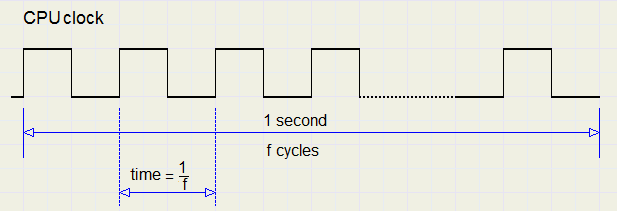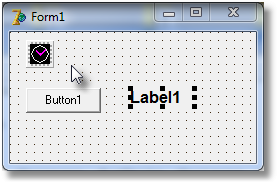a Delphi counter componentIntroduction

This article describes a microseconds counter in the Delphi (version 7) programming language.
The accuracy of this counter is in nanoseconds.
We use the internal processor clock, a 64 bit counter which counts clock cycles.
The program is organized as a Class to be installed in the Delphi component pallette.
Purpose of this project is to measure execution times.

Theory

A CPU (chip) has a certain clock rate, say 3GHz (Giga Hertz).
This means that per second 3 billion "ticks" take place.
Before converting CPU clock ticks to time, we have to find out the CPU clock frequency.

There also is a system clock, which counts milliseconds.
Function getTickCount returns this time, the number of milliseconds since power on.
However, this clock does not behave as expected.
See the program below, where subsequent increment values are written in a TMemo component:
```procedure TForm1.Button1Click(Sender: TObject);
var n : byte;
t1,t2 : dword;
s : string;
begin
memo1.Clear;
n := 0;
t1 := GetTickCount;
repeat
t2 := getTickcount;
if t2 <> t1 then
begin
inc(n);
s := inttostr(t2 - t1);
t1 := t2;
end
else application.ProcessMessages;
until n = 25;
end;

end.
```
This is the result:Milliseconds are counted, but the counter is updated only per 15 or 16 milliseconds.

Calculation of the CPU clock frequency

Using the GetTickCount we count a period of 500 milliseconds.
In this time we count the number of ticks of the 64 bit CPU clock.

To read the 64 bit CPU clock:
```function TTimer64.getCPUtime : Int64;
asm
RDTSC;  //dw \$310F
end;
```
Note: TTimer64 is the Class name. See later for details.

Note: for some reason, the following code does not work:
```function TTimer64.getCPUtime : Int64;
begin
asm
RDTSC;  //dw \$310F
end;
end;
```
The RDTSC (or hexadecimaal \$310F) command writes the lowest 32 bits in register EAX
and the highest 32 bits in register EDX.
These registers also hold function parameters of type Int64.

Calculation of the CPU clock frequency:
```constructor TTimer64.create(Aowner : TComponent);
var t1,t2 : dword;
begin
inherited create(AOwner);
t2 := getTickCount;
repeat
t1 := getTickCount;
until t2 <> t1;           //wait for millisecs counter to change
Fstart := CPUtime;
repeat
t2 := getTickCount;
until t2 - t1 >= 500;
Fend := CPUtime;
FCPUclock := (Fend - Fstart)/(t2 - t1) * 1e-3; //megahertz clock
end;
```
First we wait until the milliseconds clock increments.
CPUtime is the property that stands for function getCPUTime.
Fstart is set to the CPU clock.
Now we wait 500 milliseconds.
Then Fend is set to the CPU clock.
FCPUclock is the (floating point double) value we need.

Measuring elapsed time

Now we know the CPU clock frequency we are able to measure time accurately.
Before starting the proces we set the CPU clock in a startvalue.
After the process we place the CPU clock in an endValue. (both type Int64).
Time = (endvalue - startvalue) / clockfrequency.
Why? 1/clockfrequency = time between 2 clock cycles.
endvalue - startvalue = number of CPU ticks.The Component

```unit timercomponent;

interface
uses windows,classes,sysutils;

type TTimer64 = class(TComponent)
private
FCPUclock : double;
Fstart    : Int64;
Fend      : Int64;
protected
function getCPUclockString : string;
function getCPUtime : Int64;
function getCPUTimeString  : string;
function getElapsedTime : double;
function getElapsedTimeString : string;
public
constructor create(Aowner:TComponent);  override;
destructor destroy; override;
property CPUclock : double read FCPUclock;
property CPUclockString : string read getCPUclockString;
property CPUTimeString  : string read getCPUTimeString;
procedure start;
procedure stop;
property ElapsedTime : double read getElapsedTime;
property ElapsedTimeString : string read getElapsedTimeString;
property CPUtime : Int64 read getCPUtime;
function CPUclockToTime(I64 : Int64) : double;
end;

procedure Register;

implementation

procedure Register;
begin
RegisterComponents('system',[TTimer64]);
end;

function TTimer64.getCPUtime : Int64;
asm
RDTSC;  //dw \$310F
end;

constructor TTimer64.create(Aowner : TComponent);
var t1,t2 : dword;
begin
inherited create(AOwner);
t2 := getTickCount;
repeat
t1 := getTickCount;
until t2 <> t1;           //wait for millisecs counter to change
Fstart := CPUtime;
repeat
t2 := getTickCount;
until t2 - t1 >= 500;
Fend := CPUtime;
FCPUclock := (Fend - Fstart)/(t2 - t1) * 1e-3; //microhertz clock
end;

destructor TTimer64.destroy;
begin
inherited destroy;
end;

procedure TTimer64.Start;
begin
FStart := CPUtime;
end;

procedure TTimer64.Stop;
begin
Fend := CPUtime;
end;

function TTimer64.getElapsedTime : double;
begin
result := (Fend - Fstart) / FCPUclock;
end;

function TTimer64.CPUclockToTime(i64 : Int64) : double;
begin
result := i64 / FCPUclock;
end;

function TTimer64.getElapsedTimeString : string;
const Fconvert = '#####0.0###';
var t : double;
begin
t := getElapsedTime;
result := formatfloat(Fconvert,t);
end;

function TTimer64.getCPUclockString : string;
const Fconvert = '###0.0###';
begin
result := formatfloat(FConvert,FCPUclock);
end;

function TTimer64.getCPUTimeString : string;
var i64 : int64;
begin
i64 := CPUTime;
result := inttostr(i64);
end;

end.
```
Procedures start and stop place the CPU clock in Fstart and Fstop.
Property ElapsedTime calculates the time.
This value may also be returned as string by ElapsedTimeString.

The ancestor class is TComponent.
After installation in the component pallette a bitmap image is placed on the form,
but this image is not painted at runtime.

Application

Place a timer on the main form with name CPUt.
To display the CPU clock frequency in label1:
``` label1.caption := CPUt.CPUclockstring;
```
To pauze a program we use the comand: application.processmessages.
This returns control to Windows to process pending messages,
resulting from clicking a button or pressing a key.

We wonder how much time Windows needs.
On our main form we place a timer, TButton and TLabel component.
To the ButtonClick event we add the code:
``` with CPUt do
begin
start;
application.processmessages;
stop;
label1.caption := CPUt.ElapsedtimeString;
end;
```Just over 85 microseconds.